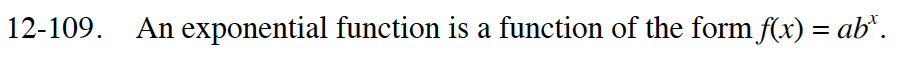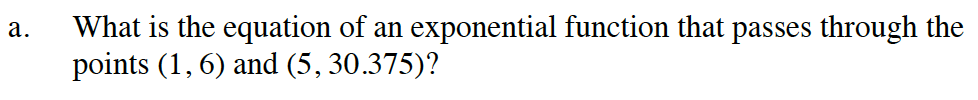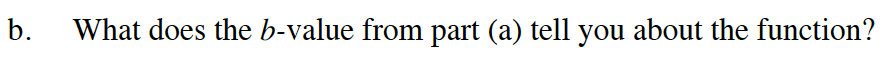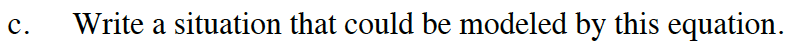### Home > INT2 > Chapter 12 > Lesson 12.2.3 > Problem12-109

12-109.
1. An exponential function is a function of the form f(x) = abx . Homework Help ✎

1. What is the equation of an exponential function that passes through the points (1, 6) and (5, 30.375)?

2. What does the b‑value from part (a) tell you about the function?

3. Write a situation that could be modeled by this equation.Substitute the points to get two equations.

6 = ab1
30.375 = ab5

Solve for a and b.

f(x) = 4(1.5)x$\text{How are the graphs of }f(x) = 2^x, f(x) = 3^x, f(x) = \left(\frac{1}{2}\right)^x \text{ different?}$Starting value should be 4.